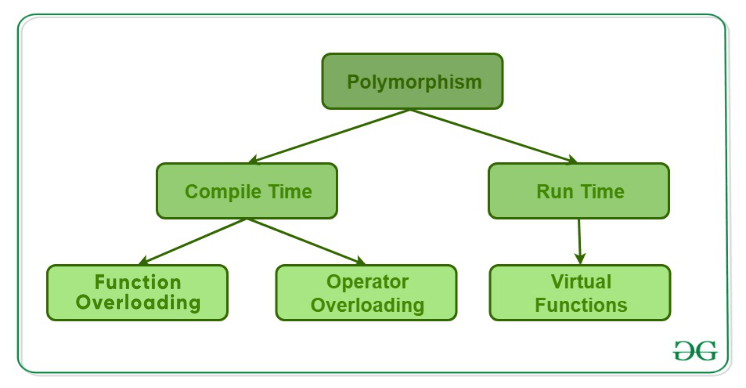# 5.1: Polymorphism in C++

$$\newcommand{\vecs}{\overset { \rightharpoonup} {\mathbf{#1}} }$$ $$\newcommand{\vecd}{\overset{-\!-\!\rightharpoonup}{\vphantom{a}\smash {#1}}}$$$$\newcommand{\id}{\mathrm{id}}$$ $$\newcommand{\Span}{\mathrm{span}}$$ $$\newcommand{\kernel}{\mathrm{null}\,}$$ $$\newcommand{\range}{\mathrm{range}\,}$$ $$\newcommand{\RealPart}{\mathrm{Re}}$$ $$\newcommand{\ImaginaryPart}{\mathrm{Im}}$$ $$\newcommand{\Argument}{\mathrm{Arg}}$$ $$\newcommand{\norm}{\| #1 \|}$$ $$\newcommand{\inner}{\langle #1, #2 \rangle}$$ $$\newcommand{\Span}{\mathrm{span}}$$ $$\newcommand{\id}{\mathrm{id}}$$ $$\newcommand{\Span}{\mathrm{span}}$$ $$\newcommand{\kernel}{\mathrm{null}\,}$$ $$\newcommand{\range}{\mathrm{range}\,}$$ $$\newcommand{\RealPart}{\mathrm{Re}}$$ $$\newcommand{\ImaginaryPart}{\mathrm{Im}}$$ $$\newcommand{\Argument}{\mathrm{Arg}}$$ $$\newcommand{\norm}{\| #1 \|}$$ $$\newcommand{\inner}{\langle #1, #2 \rangle}$$ $$\newcommand{\Span}{\mathrm{span}}$$$$\newcommand{\AA}{\unicode[.8,0]{x212B}}$$

The word polymorphism means having many forms. In simple words, we can define polymorphism as the ability of a message to be displayed in more than one form. A real-life example of polymorphism, a person at the same time can have different characteristics. Like a man at the same time is a father, a husband, an employee. So the same person posses different behavior in different situations. This is called polymorphism. Polymorphism is considered as one of the important features of Object Oriented Programming.

In C++ polymorphism is mainly divided into two types:

• Compile time Polymorphism
• Runtime Polymorphism• Function Overloading: When there are multiple functions with same name but different parameters then these functions are said to be overloaded. Functions can be overloaded by change in number of arguments or/and change in type of arguments.

// C++ program for function overloading
#include <iostream>
using namespace std;

class Junk
{
public:

// function with 1 int parameter
void func(int x)
{
cout << "value of x is " << x << endl;
}

// function with same name but 1 double parameter
void func(double x)
{
cout << "value of x is " << x << endl;
}

// function with same name and 2 int parameters
void func(int x, int y)
{
cout << "value of x and y is " << x << ", " << y << endl;
}
};

int main()
{
Junk newJunk;

// Which function is called will depend on the parameters passed
// The first 'func' is called
newJunk.func(7);

// The second 'func' is called
newJunk.func(9.132);

// The third 'func' is called
newJunk.func(85,64);
return 0;
}



Notice that the method func() is declared 3 times. The signature of each one is different - one accepts an int argument, the second accepts a float argument, and the thrid accepts 2 arguments. C++ figures out which one is to be called, based on the argument list, or upon the usage within the code.

Output:

value of x is 7
value of x is 9.132
value of x and y is 85, 64


In the above example, a single function named func acts differently in three different situations which is the property of polymorphism.

• Operator Overloading: C++ also provide option to overload operators. For example, we can make the operator (‘+’) for string class to concatenate two strings. We know that this is the addition operator whose task is to add two operands. So a single operator ‘+’ when placed between integer operands , adds them and when placed between string operands, concatenates them.
// CPP program to illustrate
#include<iostream>
using namespace std;

class Complex {
private:
int real, imag;
public:
Complex(int r = 0, int i =0) {real = r; imag = i;}

// This is automatically called when '+' is used with
// between two Complex objects
Complex operator + (Complex const &obj) {
Complex res;
res.real = real + obj.real;
res.imag = imag + obj.imag;
return res;
}
void print() { cout << real << " + i" << imag << endl; }
};

int main()
{
Complex c1(10, 5), c2(2, 4);
Complex c3 = c1 + c2; // An example call to "operator+"
c3.print();
}


Output:

12 + i9


In the above example the operator ‘+’ is overloaded. The operator ‘+’ is an addition operator and can add two numbers(integers or floating point) but here the operator is made to perform addition of two imaginary or complex numbers. To learn operator overloading in details visit this link.

Runtime polymorphism: This type of polymorphism is achieved by Function Overriding.

• Function overriding on the other hand occurs when a derived class has a definition for one of the member functions of the base class. That base function is said to be overridden.
// C++ program for function overriding
#include <iostream>
using namespace std;

class base
{
public:
virtual void print ()
{
cout<< "print in base class" <<endl;
}

void show ()
{
cout<< "show in base class" <<endl;
}
};

class derived:public base
{
public:
// print() is already virtual function in
// derived class, we could have declared
// it as virtual void print() explicitly
void print ()
{
cout<< "print in derived class" <<endl;
}

void show ()
{
cout<< "show in derived class" <<endl;
}
};

//main function
int main()
{
base *bptr;
derived d;
bptr = &d;

//virtual function, bound at runtime (Runtime polymorphism)
bptr->print();

// Non-virtual function, bound at compile time
bptr->show();

return 0;
}


The idea is that virtual functions are called according to the type of the object instance pointed to or referenced, not according to the type of the pointer or reference. This means that in this example, bptr is pointing at a derived type, &d, so therefore at execution time we run the derived class print() method, because the base class print() method is virtual. Since the base class show() method is NOT virtual, then we run the base class show() method.

Output:

print in derived class
show in base class


We will take a closer look at this example on the next page.